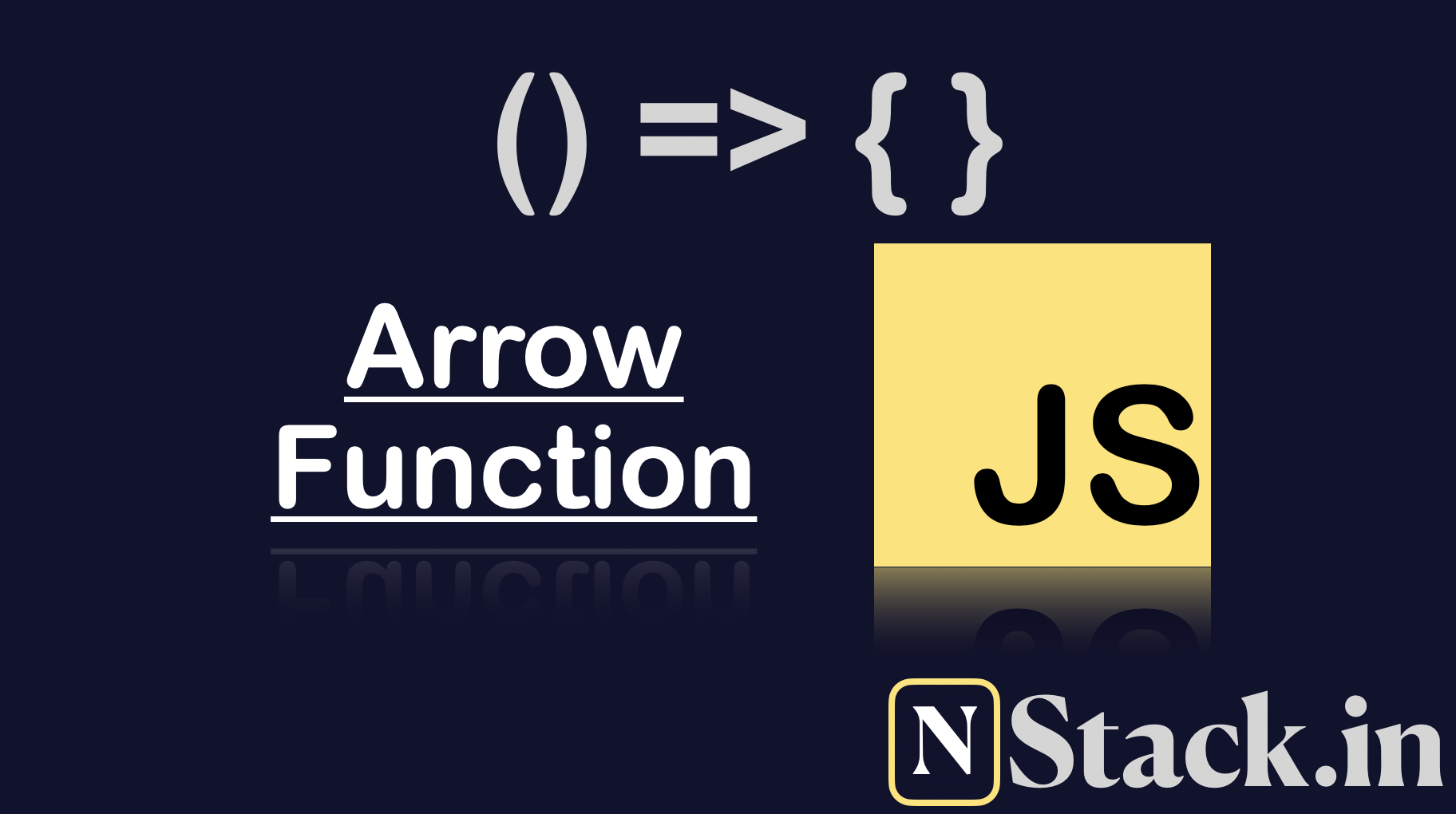Nitish Kumar Singh9 minutes
Posted on
September 28, 2020

# JavaScript fat arrow function

Those of you heard this term `fat arrow function` or `arrow function` for first time then let me tell you one thing it’s just syntactic sugar.

I will compare all the common possibility and try to make you a better developer. So, you will not get caught by any arrow function related bug in near future.

## Basics #

Let’s talk about some basics before getting into some new concept `arrow function`. There are two popular way of defining the function in javascript and you must know about this before getting into arrow function.example-1.js
``````// Function 1 : Function with one statement
function sum(num1, num2) {
return num1 + num2;
}

// Function 2 : Function with one statement
var add = function (num1, num2) {
return num1 + num2;
};
``````

The above example is simple and it’s okay for most of you but there is bit possibility that some of you might not know about the second ways.

Both function have some different behaviors and those who don’t know let me tell you that.

`Function 1` is hoisted and `Function 2` isn’t. Hosting means you can call the function before defining and it will work.

Hoisting concept is also somewhere related to arrow function, so you must know about that before getting into arrow function.

## Hoisting #

Let’s understand the hosting by example because code speaks louder. Seeing the code you will get much more understanding than whatever I write or speak.hoisting-demo-1.js
``````var result = sum(2, 3);
console.log(result); // 5

function sum(num1, num2) {
return num1 + num2;
}
``````

The above example is correct and if you will run this, it should work. We are calling the sum function before declaring/defining but that’s fine because function it’s what hoisting is.

Let see one other example which isn’t hoisted and get the clear understanding about hoisting.hoisting-demo-2.js
``````var result = add(2, 3);
console.log(result); // Uncaught TypeError: add is not a function

var add = function (num1, num2) {
return num1 + num2;
};
``````

We can’t call `add` function before we assign

## Writing first arrow function #

Let’s write our first arrow function and run it. There is two way of writing arrow function and both have different use case.example-1-arrow.js
``````// Function 3 : Arrow Function are mostly written like this
var print = () => {
console.log("My name is print");
};

// Function 4 : Arrow function can also be written like this
var printing = () => console.log("My name is printing");
``````
• Arrow function can’t be hoisted, which means you need to define it before using it.

Sometimes we want to write the funtion without the argumnents.

``````var quad = (_) => console.log("I do not have any arguments");
``````

## Example 2 #

`Arrow function` with multiple statement can’t be written in single line like a statement.

This example will cover `function` with more than one statementexample-2.js
``````// Function with more than one statement
function cube(num) {
var result = num * num;
return result;
}

// Function with more than one statement
var cube = function (num) {
var result = num * num;
return result;
};

// Function with more than one statement
var cube = (num) => {
var result = num * num;
return result;
};
``````

## Example 3 #

In this example you will see the difference between arrow and regular function.

I know after seeing the above examples, you might say both are same. You can easily convert any `function` to `arrow function` by doing doing some syntax tweaks.example-3.js
``````// Regular function
function threeOne() {
console.log(this);
}

// Arrow function
var threeTwo = () => {
console.log(this);
};

// Arrow function
var threeThree = () => console.log(this);
``````

try the above code in your console and you will find different result. You can clearly see that i am printing this in all the function.

Now you see some difference but now you are curious , is this different is important for us.

You can think this difference is not going to make our life different because we don’t write any code using `this`.

Yes we face this kind of situation a lot, but if you write javascript more. You might have also faced this issue if you have written code in react and vue.

## Example 4 #

In this example I will demonstrate the above problem in real life and it’s solution.example-4.js
``````//
``````
Last updated at Monday, Sep 7, 2020 by Nitish Kumar Singh
comments powered by Disqus